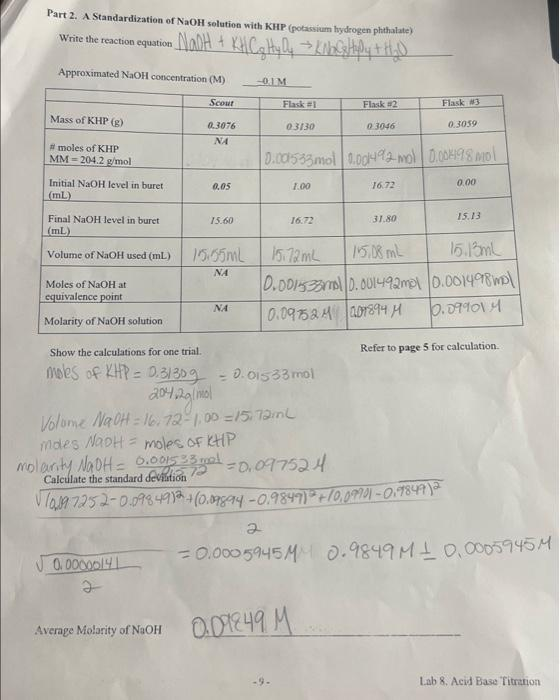Home / Expert Answers / Chemistry / part-2-a-standardization-of-naoh-solution-with-khp-potassium-hydrogen-phthalate-approximated-naoh-pa490

# (Solved): Part 2. A Standardization of NaOH solution with KHP (potassium hydrogen phthalate) Approximated NaOH ...

Part 2. A Standardization of NaOH solution with KHP (potassium hydrogen phthalate) Approximated NaOH concentration (M)= ~0.1 Mass of KHP (g) #moles of KHP MM 204.2 g/mol = Initial NaOH level in buret (mL) Final NaOH level in buret (mL) Volume of NaOH used (mL) Moles of NaOH at equivalence point Molarity of NaOH solution Scout 0.00000141 2 0.3076 ?? Average Molarity of NaOH 0.05 15.60 15.55mL ?? ?? ~0.1 M Flask #1 0.3130 Show the calculations for one trial. moles of KHP = 0.31309 204,2g/mol Volume NaOH = 16,72-1,00 =15,72ml 1.00 0.001533mol 0.001492 mol 0.001498 mol 16.72 0.01533mol -9- Flask #2 0.09249 M 0.3046 15172mL 15,08 mL 0.001533ml 0.001492mol 0.091524 09894 M 16.72 31.80 moles NaOH = moles of KHP molarity NaOH = 0.001533 mol Calculate the standard deviation 72 =0,097524 ? 10,897252-0.09849)2 + (0,09894 -0.9849)2 + 10,09901 -0,9849) 2 Flask #3 0.3059 0.00 15.13 15.13ml 0.001498 mol 10.09901M Refer to page 5 for calculation. 2 -0.0005945MM 0.9849M 1 0.0005945M Lab 8. Acid Base TitrationPart 2. A Standardization of solution with KHP (potassium hydrogen phthalate) Approximated NaOH concentration (M) Show the calculations for one trial. Refer to page 5 for calculation.

We have an Answer from Expert